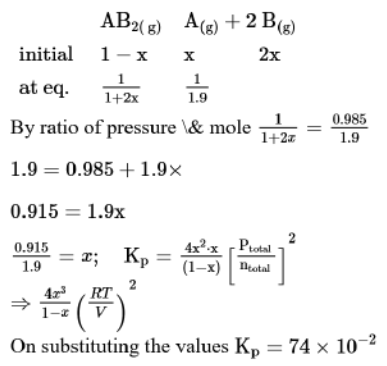# A homogeneous ideal gaseous reaction

Question:

A homogeneous ideal gaseous reaction $\mathrm{AB}_{2(\mathrm{~g})} \rightleftharpoons \mathrm{A}_{(\mathrm{g})}+2 \mathrm{~B}_{(\mathrm{g})}$ is carried out in a 25 litre flask at $27^{\circ} \mathrm{C}$. The initial amount of $\mathrm{AB}_{2}$ was 1 mole and the equilibrium pressure was $1.9$ atm. The value of $K_{p}$ is $x \times 10^{-2}$. The value of $x$ is ______________$\quad\left[R=0.08206 \mathrm{dm}^{3} \mathrm{~atm} K^{-1} \mathrm{~mol}^{-1}\right]$

Solution:

(74)